# RD Sharma Solutions for Class 9 Maths Chapter 25 Probability

## RD Sharma Solutions Class 9 Maths Chapter 25 – Free PDF Download

RD Sharma Solutions for Class 9 Maths Chapter 25 Probability are given here which consists of questions and answers related to Probability. “Probability is a measure of the possibility that an event will occur”. It is qualified as a number between zero and one. A simple example of probability is the tossing of the coin. A coin consists of two sides, a head and a tail, which means there are only two outcomes. The probability of tails equals the probability of heads. The probability of tails or heads is 1/2 since there are no other outcomes. The comprehensive answers present in RD Sharma Solutions boosts confidence to solve any type of problem in an efficient manner.

What is Probability?

Probability is a measure of the likelihood of an event to occur. Many events cannot be predicted with total certainty. We can predict only the chance of an event to occur, how likely they are to happen, using probability. Practice more Maths concepts following RD Sharma Class 9 Solutions designed by experts in accordance with CBSE syllabus and score good marks in exams.

## Download PDF of RD Sharma Solutions for Class 9 Maths Chapter 25 Probability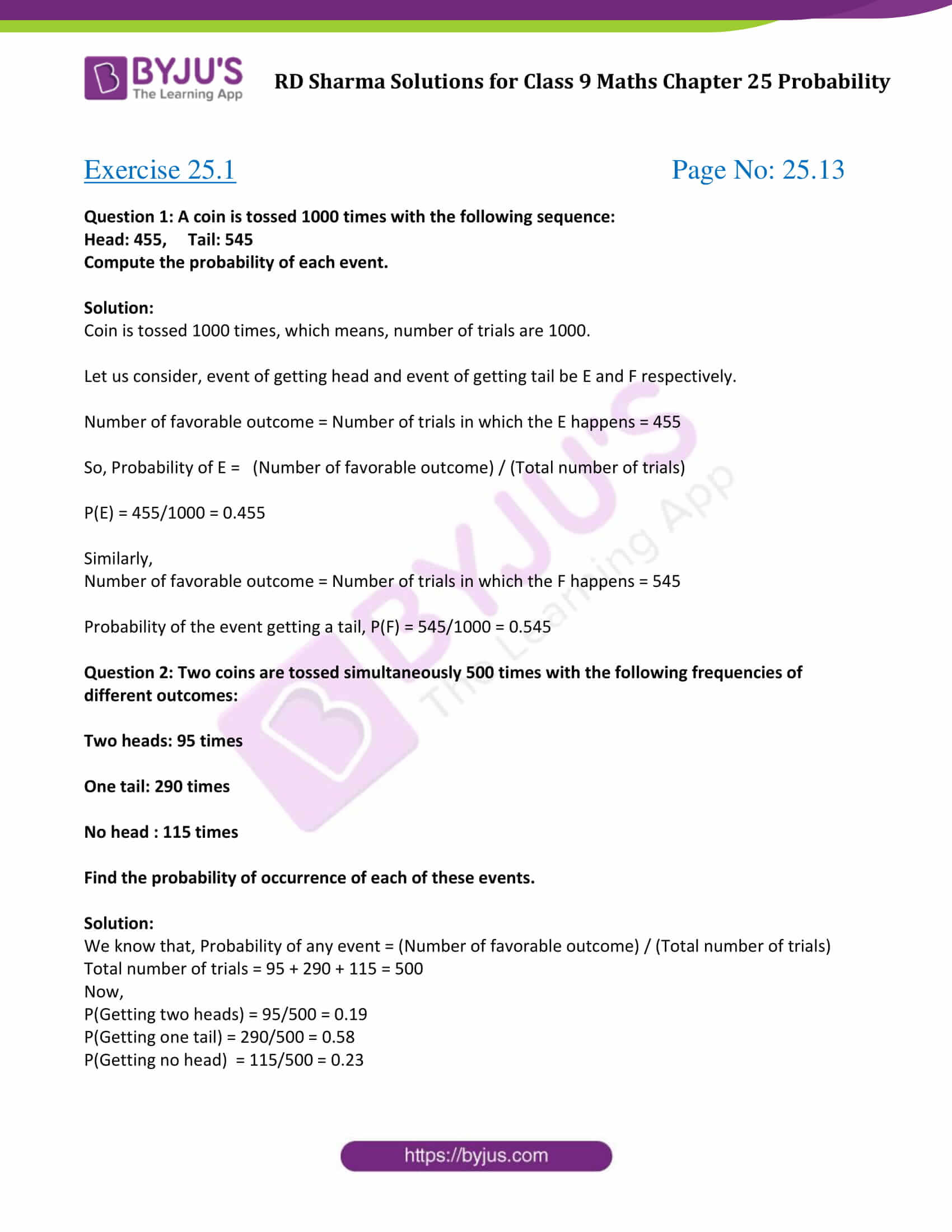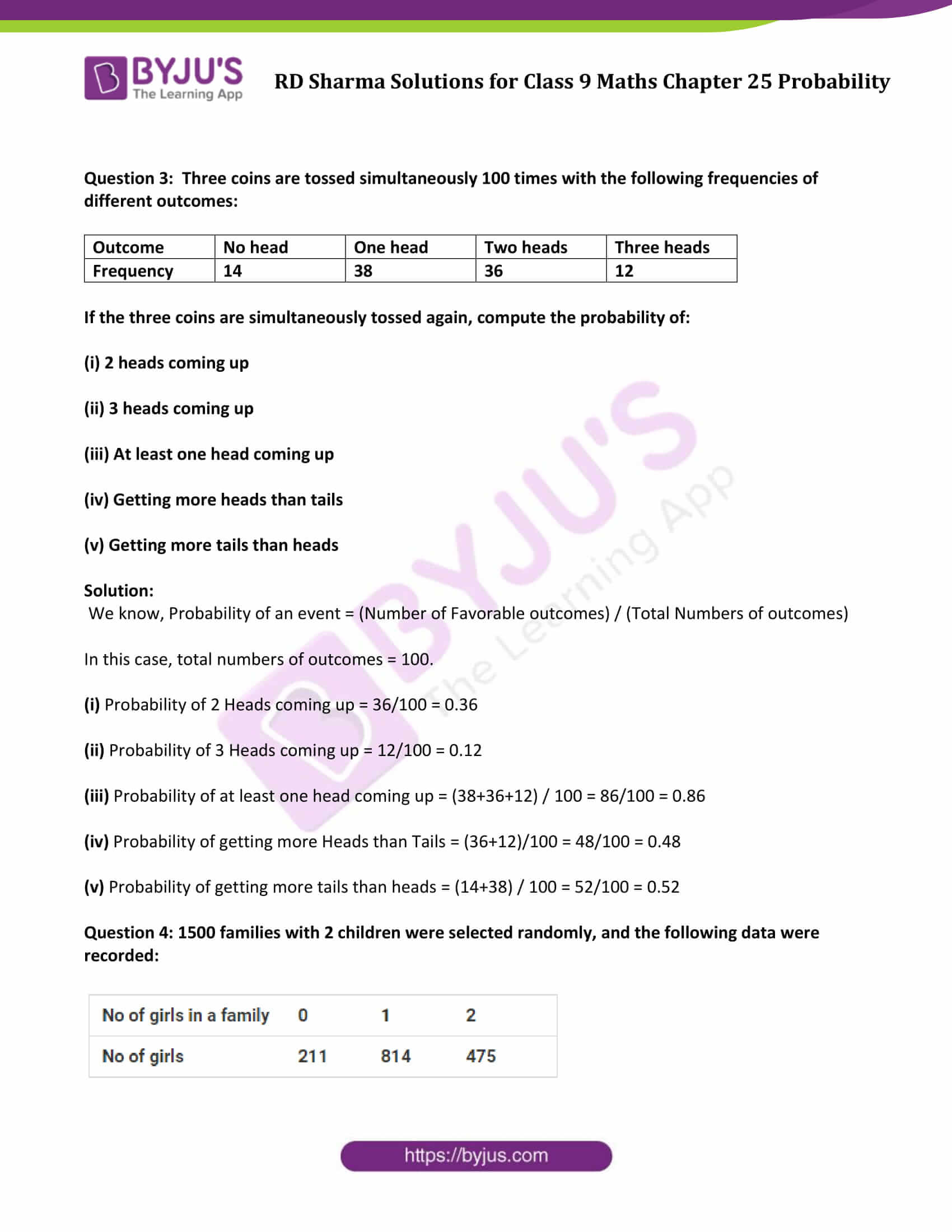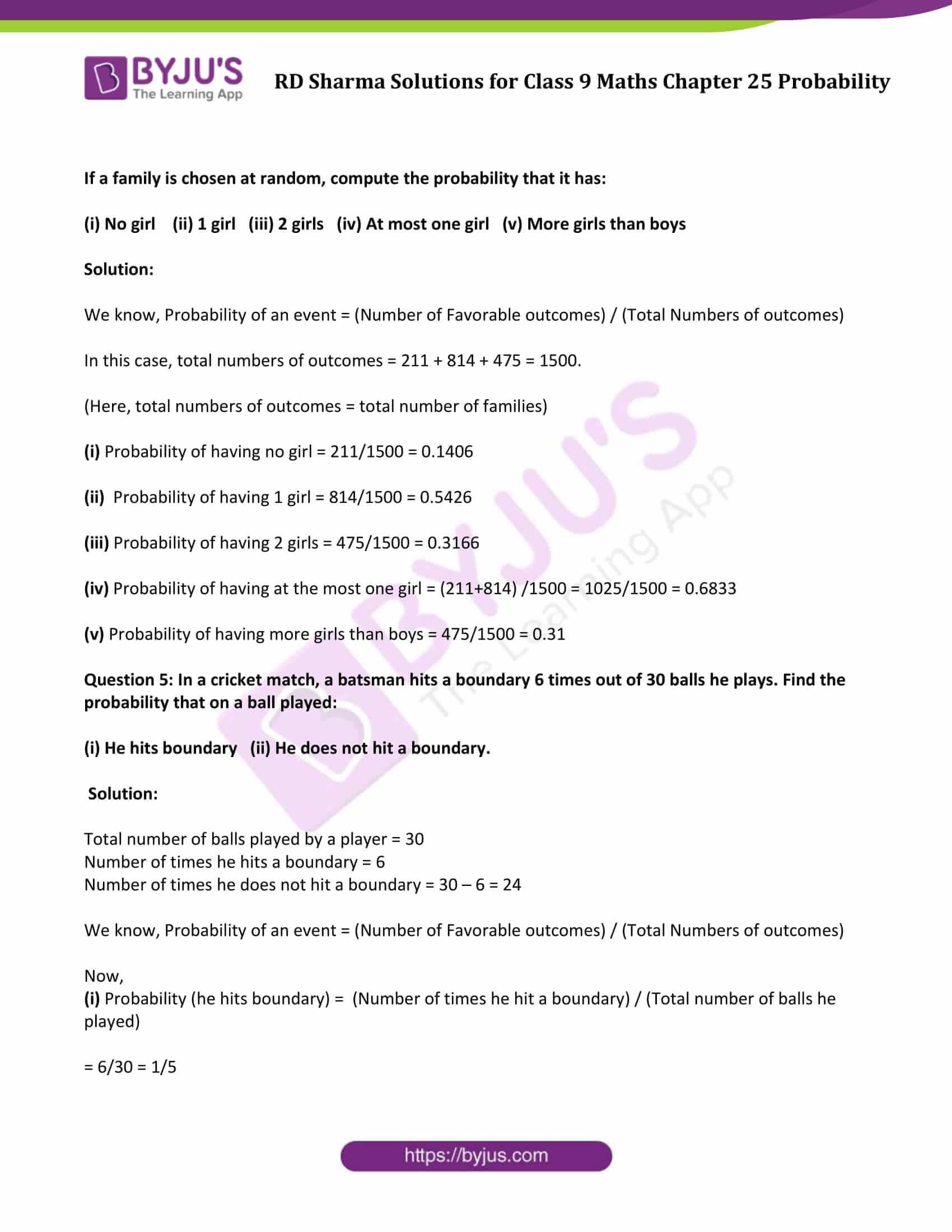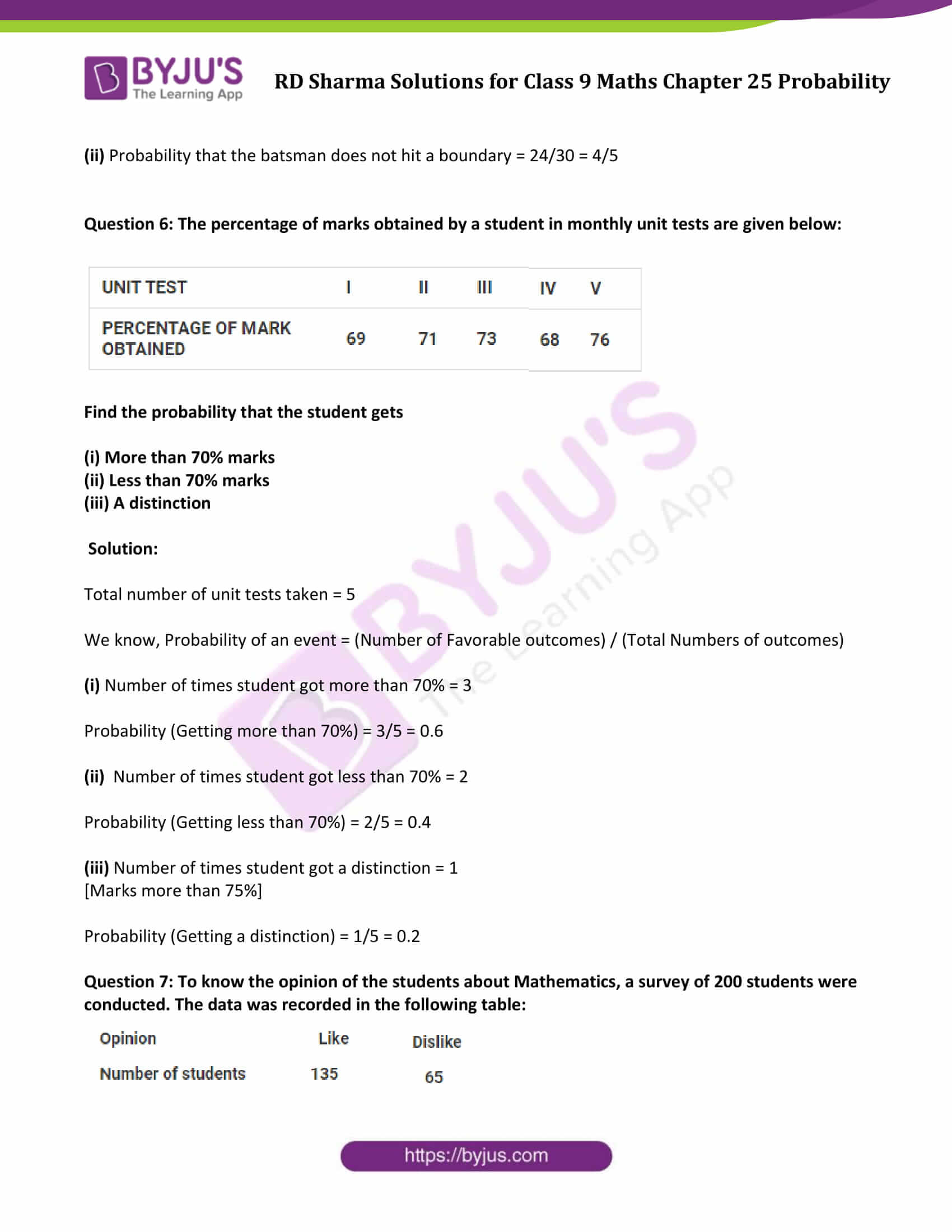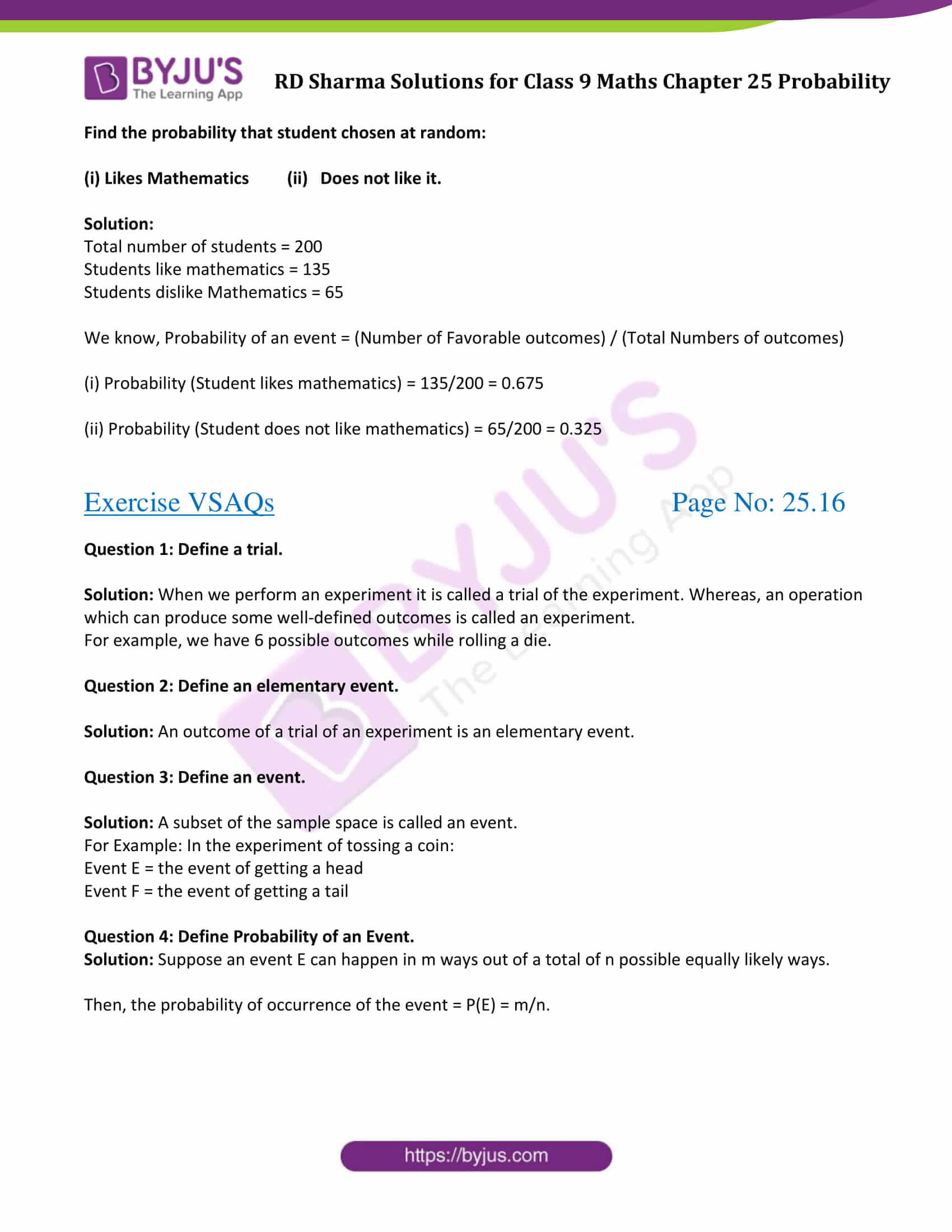### Access Answers to Maths RD Sharma Solutions for Class 9 Chapter 25 Probability

Exercise 25.1 Page No: 25.13

Question 1: A coin is tossed 1000 times with the following sequence:

Compute the probability of each event.

Solution:

Coin is tossed 1000 times, which means, number of trials are 1000.

Let us consider, event of getting head and event of getting tail be E and F respectively.

Number of favorable outcome = Number of trials in which the E happens = 455

So, Probability of E = (Number of favorable outcome) / (Total number of trials)

P(E) = 455/1000 = 0.455

Similarly,

Number of favorable outcome = Number of trials in which the F happens = 545

Probability of the event getting a tail, P(F) = 545/1000 = 0.545

Question 2: Two coins are tossed simultaneously 500 times with the following frequencies of different outcomes:

One tail: 290 times

Find the probability of occurrence of each of these events.

Solution:

We know that, Probability of any event = (Number of favorable outcome) / (Total number of trials)

Total number of trials = 95 + 290 + 115 = 500

Now,

P(Getting two heads) = 95/500 = 0.19

P(Getting one tail) = 290/500 = 0.58

P(Getting no head) = 115/500 = 0.23

Question 3: Three coins are tossed simultaneously 100 times with the following frequencies of different outcomes:

If the three coins are simultaneously tossed again, compute the probability of:

(iii) At least one head coming up

(iv) Getting more heads than tails

(v) Getting more tails than heads

Solution:

We know, Probability of an event = (Number of Favorable outcomes) / (Total Numbers of outcomes)

In this case, total numbers of outcomes = 100.

(i) Probability of 2 Heads coming up = 36/100 = 0.36

(ii) Probability of 3 Heads coming up = 12/100 = 0.12

(iii) Probability of at least one head coming up = (38+36+12) / 100 = 86/100 = 0.86

(iv) Probability of getting more Heads than Tails = (36+12)/100 = 48/100 = 0.48

(v) Probability of getting more tails than heads = (14+38) / 100 = 52/100 = 0.52

Question 4: 1500 families with 2 children were selected randomly, and the following data were recorded: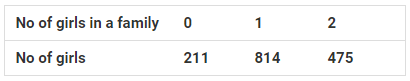If a family is chosen at random, compute the probability that it has:

(i) No girl (ii) 1 girl (iii) 2 girls (iv) At most one girl (v) More girls than boys

Solution:

We know, Probability of an event = (Number of Favorable outcomes) / (Total Numbers of outcomes)

In this case, total numbers of outcomes = 211 + 814 + 475 = 1500.

(Here, total numbers of outcomes = total number of families)

(i) Probability of having no girl = 211/1500 = 0.1406

(ii) Probability of having 1 girl = 814/1500 = 0.5426

(iii) Probability of having 2 girls = 475/1500 = 0.3166

(iv) Probability of having at the most one girl = (211+814) /1500 = 1025/1500 = 0.6833

(v) Probability of having more girls than boys = 475/1500 = 0.31

Question 5: In a cricket match, a batsman hits a boundary 6 times out of 30 balls he plays. Find the probability that on a ball played:

(i) He hits boundary (ii) He does not hit a boundary.

Solution:

Total number of balls played by a player = 30

Number of times he hits a boundary = 6

Number of times he does not hit a boundary = 30 – 6 = 24

We know, Probability of an event = (Number of Favorable outcomes) / (Total Numbers of outcomes)

Now,

(i) Probability (he hits boundary) = (Number of times he hit a boundary) / (Total number of balls he played)

= 6/30 = 1/5

(ii) Probability that the batsman does not hit a boundary = 24/30 = 4/5

Question 6: The percentage of marks obtained by a student in monthly unit tests are given below: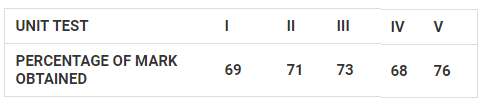Find the probability that the student gets

(i) More than 70% marks

(ii) Less than 70% marks

(iii) A distinction

Solution:

Total number of unit tests taken = 5

We know, Probability of an event = (Number of Favorable outcomes) / (Total Numbers of outcomes)

(i) Number of times student got more than 70% = 3

Probability (Getting more than 70%) = 3/5 = 0.6

(ii) Number of times student got less than 70% = 2

Probability (Getting less than 70%) = 2/5 = 0.4

(iii) Number of times student got a distinction = 1

[Marks more than 75%]

Probability (Getting a distinction) = 1/5 = 0.2

Question 7: To know the opinion of the students about Mathematics, a survey of 200 students were conducted. The data was recorded in the following table: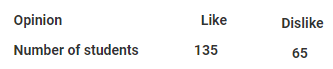Find the probability that student chosen at random:

(i) Likes Mathematics (ii) Does not like it.

Solution:

Total number of students = 200

Students like mathematics = 135

Students dislike Mathematics = 65

We know, Probability of an event = (Number of Favorable outcomes) / (Total Numbers of outcomes)

(i) Probability (Student likes mathematics) = 135/200 = 0.675

(ii) Probability (Student does not like mathematics) = 65/200 = 0.325

Exercise VSAQs Page No: 25.16

Question 1: Define a trial.

Solution: When we perform an experiment it is called a trial of the experiment. Whereas, an operation which can produce some well-defined outcomes is called an experiment.

For example, we have 6 possible outcomes while rolling a die.

Question 2: Define an elementary event.

Solution: An outcome of a trial of an experiment is an elementary event.

Question 3: Define an event.

Solution: A subset of the sample space is called an event.

For Example: In the experiment of tossing a coin:

Event E = the event of getting a head

Event F = the event of getting a tail

Question 4: Define Probability of an Event.

Solution: Suppose an event E can happen in m ways out of a total of n possible equally likely ways.

Then, the probability of occurrence of the event = P(E) = m/n.

## RD Sharma Solutions for Class 9 Maths Chapter 25 Probability

In the 25th Chapter of Class 9 RD Sharma Solutions  students will study important concepts listed below:

• Probability Introduction
• Various approaches to Probability
• Experimental or empirical approach to Probability
• Some important terms: Trial, Elementary event and Compound event

## Frequently Asked Questions on RD Sharma Solutions for Class 9 Maths Chapter 25

### Where can I get the accurate answers for RD Sharma Solutions for Class 9 Maths Chapter 25?

At BYJU’S, you can get the accurate answers in PDF format for RD Sharma Solutions for Class 9 Maths Chapter 25. The solutions for each textbook question for Chapter 25 have been designed precisely by Mathematics experts at BYJU’S. The solutions are explained in a simple and lucid manner as per the current CBSE syllabus.

### Is it important to practice all the questions provided in RD Sharma Solutions for Class 9 Maths Chapter 25?

Yes, since all types of questions are vital from an exam perspective, you must practice all of them. Practising all the questions on a daily basis help students to grasp the concepts flawlessly and score good marks in exams. With the aim of providing the best source for students, our expert faculty have prepared the solutions accurately in a simple language.

### Mention the important topics covered in RD Sharma Solutions for Class 9 Maths Chapter 25

The important topics covered in RD Sharma Solutions for Class 9 Maths Chapter 25 are given below:

1. Probability Introduction
2. Various approaches to Probability
3. Experimental or empirical approach to Probability
4. Some important terms: Trial, Elementary event and Compound event